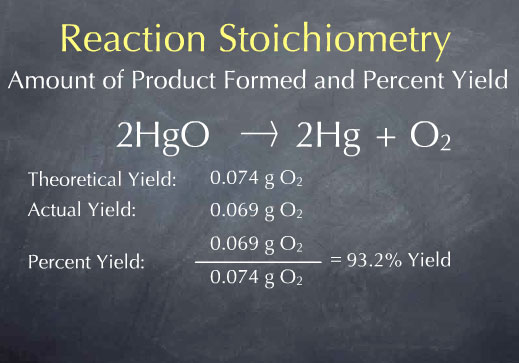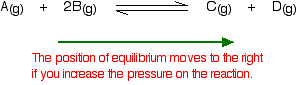## Tuesday, October 23, 2012

### Mole Day 2012

Today is Mole Day! Mole day celebrates Avogadro's number of 6.02 x 10 to the 23rd power a ginormous number that helps us keep track of itty bitty atoms.

Students learned about moles way back in Unit 1 and already know the basics. We have been honing those skills all along - especially since starting Unit 5 Stoichiometry.

For moles there are basically four  conversions to know.

1 mole
6.02 x 10^23

1 mole
(molar mass) grams (add weights from PT)

1 mole
22.4 Liters

Mole
Mole

The mole/mole conversion is used to convert from one chemical to another using the coefficients from a balanced equation.

Students came up with team names related to moles and designed team flags. Then teams started in on the Avogadro Cup with silly activities related to moles (both animal moles and scientific moles) like "Pin the Nose on the Mole" and "Mole Celebrities" and the "Periodic Table Puns." Students also did CheMOLEstry activities like mole conversions and practice SOL questions.

## Friday, October 19, 2012

### Theoretical and Percent Yield

Theoretical yield is how much product you can make with given reactants. To determine theoretical yield, do a normal stoichiometry calculation.Actual yield is how much is actually produced when the reaction is performed.

Percent yield is how well you do. Percent yield calculates how close to the theoretical yield you are. A high percent yield means that your actual yield was close to the theoretical yield, the reaction worked the way it was supposed to it, and it was efficient and accurate.

Why doesn't theoretical yield equal actual yield very often? ERROR!

Error can include impure substances, uncalibrated equipment, improper procedure... all kinds of things.

Percent error measures the amount of error. Small percent error means things went well and the actual yield was close to the theoretical yield.

Percent yield plus percent error should equal 100.

## Wednesday, October 17, 2012

### Limiting Reactants

If you mix two chemicals together as reactants it is unlikely that both reactants will be used up completely when forming products. One will be used to completion, this one is the limiting reactant, and will limit how much product can be made. The other will be used up until the other reactant runs out and there will be some left over, so it is an excess reagent.

If you have 8 cars and 48 tires, which one is limiting? Which one will you run out of first?

In this example it is easy to "see" which is the limiting and which is excess. When looking at quantities of chemicals it is not as easy to "see."

To determine which chemical is limiting, convert from the given reactants to a product (it does not matter which). Whichever reactant produces the least amount of product is limiting.

4 NH3(g) + 5 O2(g)4 NO(g) + 6 H2O(g)

If you have 2.00 grams of ammonia and 4.00 grams of oxygen gas which is limiting?
Because the oxygen produced less of the product nitrogen monoxide, oxygen is limiting.

## Monday, October 15, 2012

### Stoichiometry

Stoichiometry is the most important part of chemistry and why chemistry is so useful in real life. With a balanced equation, stoichiometric conversions can be used to calculate how much product will be made, or how much reactant is needed to produce a certain amount of product.

Stoichiometry uses the three mole conversions that students are familiar with from unit one, plus the mole/mole conversion. A mole/mole conversion uses the coefficients from a balanced equation to convert from one chemical to another. You can only compare elements or chemicals when they are both in mole form.

Using this equation N2 + 3 H2 --> 2 NH3 the following calculations can be made using stoichiometry.

## Wednesday, October 10, 2012

### Entropy - Disorder

Entropy is a chemistry word for disorder. An increase in entropy is spontaneous. By looking for four things in a reaction, students can determine whether a reaction is spontaneous or nonspontaneous by looking for an increase in entropy.

Exothermic reactions are spontaneous and show an increase in entropy.

Gases are messier than solids, so a reaction that forms a gas shows an increase in entropy.

More molecules show an increase in entropy. Count the coefficients on either side of a balanced equation. If the products have more molecules then there is an increase in entropy and the reaction could be spontaneous.

A decrease in the size of molecules (count atoms making up the molecule) is an increase in entropy.

Students look for all four things and decide whether the overall reaction would lead to an increase in entropy and be spontaneous.

## Monday, October 8, 2012

### Le Chatlier

Students learned about reaction rates and how to increase them. They also learned about reversible reactions and how Le Chatlier's principle influences shifts of equilibrium in reversible reactions.

Basically as you apply a stress to a system, the system will shift in response to the stress. If you add one of the molecules it will shift away from that molecule. If you take away a molecule, it will shift towards it to make more. Heat works the same way.Pressure is the tricky one. If pressure is applied to an equilibrium, then the reaction will shift to the side that has the least amount of molecules (count the coefficients).

## Friday, October 5, 2012

### Reaction Rate Basics

Reaction Rates are affected by a few things. Without telling them the point, the students had a quick demo where they had to dissolve sugar cubes the fastest.

The things that speed up reactions are:
• Temperature - warmer is faster
• Surface Area - small pieces have more surface area
• Concentration - the more water, the faster sugar will dissolve
• Catalyst - lowers the activation energy and speeds up the reaction
• Agitation - shaking or stirring increases the frequency of collisions.

## Monday, October 1, 2012

### Balancing Reactions

Students are learning to balance equations. Today they learned that reactants are what you start with and are on the left side of the equation. Products are on the right side of the arrow and are what is made by process of a chemical change.

Because of the Law of Conservation of Mass, the number of atoms have to be equal on both sides. To balance an equation, the coefficients are changed. Coefficients are the big numbers in front that tell you how many molecules there are. The subscripts (the little lower numbers) are not allowed to be changed because those are there to make neutrally bonded molecules (what we learned in the last unit.

By changing the coefficients and counting the number of atoms on both sides of the arrow, balancing can be achieved.